Special cases of triangle congruence
in free fall periodic orbits

12 cases with unit masses
...with masses 1, 0.8, 0.8

By Alexander Gofen (February 2022)

Below we introduce a gallery of 12 orbits of the 30 cases of periodic "free fall" in the 3-body problem (*) having unit masses:
"free fall" meaning that the initial velocities in all 3 masses are zero. All 30 cases present periodic motion with a particular periods T, and their numbering follows the table of the initial values in (*).

(*). Xiaoming Li and Shijun Liao, Movies of the Collisionless Periodic Orbits in the Free-fall Three-body Problem in Real Space or on Shape Sphere
http://numericaltank.sjtu.edu.cn/free-fall-3b/free-fall-3b-movies.htm

The selected 12 simulations are special in that the initial triangle formation of rest at t=0 happens to be congruent to the second triangle formation of rest at the moment t=T/2. The moments of rest (
0 and T/2) will be also referred as the break points.

The congruent triangles are plotted in gray.

Of those 12 congruencies, there are 9 cases where the
triangles in the pairs relate via reflection, and 3 cases when the triangles in the pairs are in central symmetry.

The table of all the 30 orbits

Here we summarize the newly discovered properties in 12 of the 30 orbits. The Table 1 below shows in which of the 30 simulations (*) these earlier unknown properties take place.

We consider two triangular formations of the 3 bodies: the initial △ABC at the moment t=0 and the second △A′B′C′ at the moment t=T/2, where △ABC and △A′B′C′  are congruent: with or without reflection. This means the equality of the corresponding angles ∠A=∠A′,  ∠B=∠B′,  ∠C=∠C′.

Let the bodies
#1, #2, #3 at the initial moment reside correspondingly at the vertices A, B, C of the △ABC. Their trajectories, however, may not necessarily lead to the corresponding vertices A′, B′, C′ of the △A′B′C′, as some of the simulations below demonstrate. Among the data collected by the special research program, there are the permutations (αβγ), where the identity permutation is denoted  Id = (123). In the case of the Id  permutation, the trajectories of the bodies #1, #2, #3 lead from the vertices A, B, C to the corresponding vertices A′, B′, C′ (no matter whether △A′B′C′ is a reflection of △ABC or not).

These newly discovered properties in the 12 of the 30 follow below (the numbering begins with 3 in order to match the numbering in this article).

3. In the moments (1/4)T and (3/4)T the bodies are either in syzygy (Case 5), or they form an isosceles triangle (Case 6).

4. The triangle formation at the second moment of rest (1/2)T is congruent to the initial triangle.

5. In 3 of the 12 orbits in the moments (1/4)T and (3/4)T the second triangle is a result of 180˚ rotation of the initial triangle so that both triangles and respective parts of orbits are symmetric over the central point lying on the syzygy, one of the bodies being in the middle. At that, the three vectors of the velocities in the moments (1/4)T and (3/4)T are reciprocally parallel. However...

6. In the remaining 9 orbits the edges of the triangles in pairs are not parallel as both triangles are in the relation of reflection, i.e. the two respective parts of orbits are symmetric over some line of symmetry which, however, is not necessarily the line of syzygy. Specifically...

7. If there is no permutation (i.e. Id takes place), then in the moments T/4 and (3/4)T the 3 bodies are in syzygy on the line of symmetry, otherwise in the moments T/4 and (3/4)T the bodies are not in syzygy forming an isosceles triangle (Robert Montgomery).

 At  t = T/2 At  t = T/4 # Congruency Parallel edges Symmetry Permutation Isosceles Syzygy 1 2 3 4 Yes Reflection (321) Yes 5 6 Yes Reflection (321) Yes 7 8 Yes Reflection Id Yes 9 10 Yes Reflection Id Yes 11 12 13 14 Yes Yes Central (132) Yes Yes 15 Yes Yes Central (132) Yes Yes 16 17 18 Yes Reflection Id Yes 19 Yes Reflection (213) Yes 20 21 22 Yes Reflection Id Yes 23 24 25 Yes Reflection Id Yes 26 27 Yes Yes Central (213) Yes Yes 28 29 Yes Reflection Id Yes 30

How the triplets of initial points were obtained

As explained in (*), the authors fixed the points q1 = (-0.5, 0),  q2 = (0.5, 0),  while the goal of the search algorithm was to obtain points q3 such that the target function be near zero with the specified accuracy. (The target function was defined so that it reaches zero when a period is found). Below is a scattered graph for the 30 points q3 obtained in the search process (*):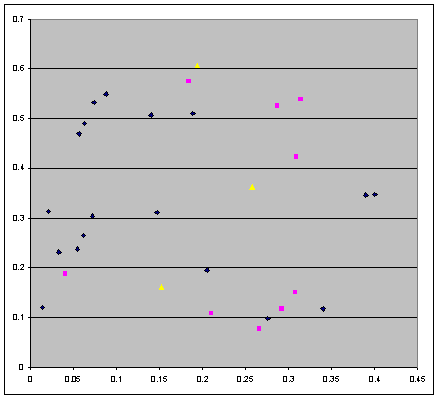Figure 1. The 30 values for the third initial point q3 obtained in a search algorithm

The three yellow points correspond to the cases of the central symmetry, the 9 magenta points correspond to the cases of reflection, and the remaining blue points correspond to the orbits with no special properties. This graph does not reveal any remarkable pattern. There is no mentioning in
(*) whether the search algorithm delivered all existing points q3 in the given bounded area of search, and whether the number of points q3 is finite or infinite.

9 cases of congruence with reflection
In these 9 cases of congruence with reflection, the two triangles are in the relation of reflection over a certain line of symmetry (in yellow), and also the entire trajectories have the same line of symmetry. In some of the following 9 cases with reflection, a non-Id permutation takes place, meaning that the trajectory of a body #i (1 ≤ i ≤3) starting at a vertex X reaches a vertex Y X,  X, Y ∈ {A, B, C}. If this is the case, the geometrically respective vertices would have different colors in accordance with the moving body color.

With a
non-Id permutation, at the moments T/4 and 3T/4 the 3 bodies are not in syzygy (for example, simulation 4) making an isosceles formation instead.

Otherwise, in the case of the
Id, at the moments T/4 and 3T/4 the 3 bodies are in syzygy which is also the line of symmetry (for example, simulation 8).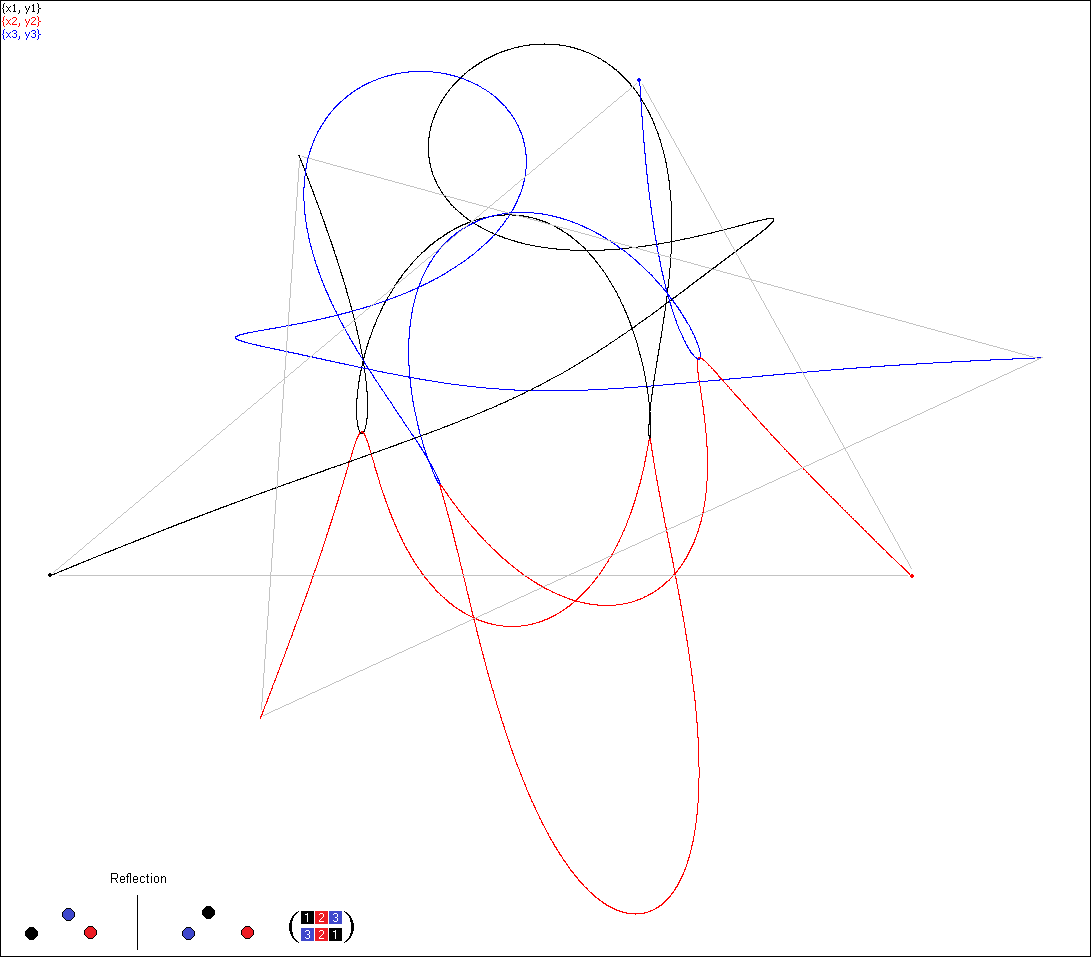Simulation 4, permutation (321)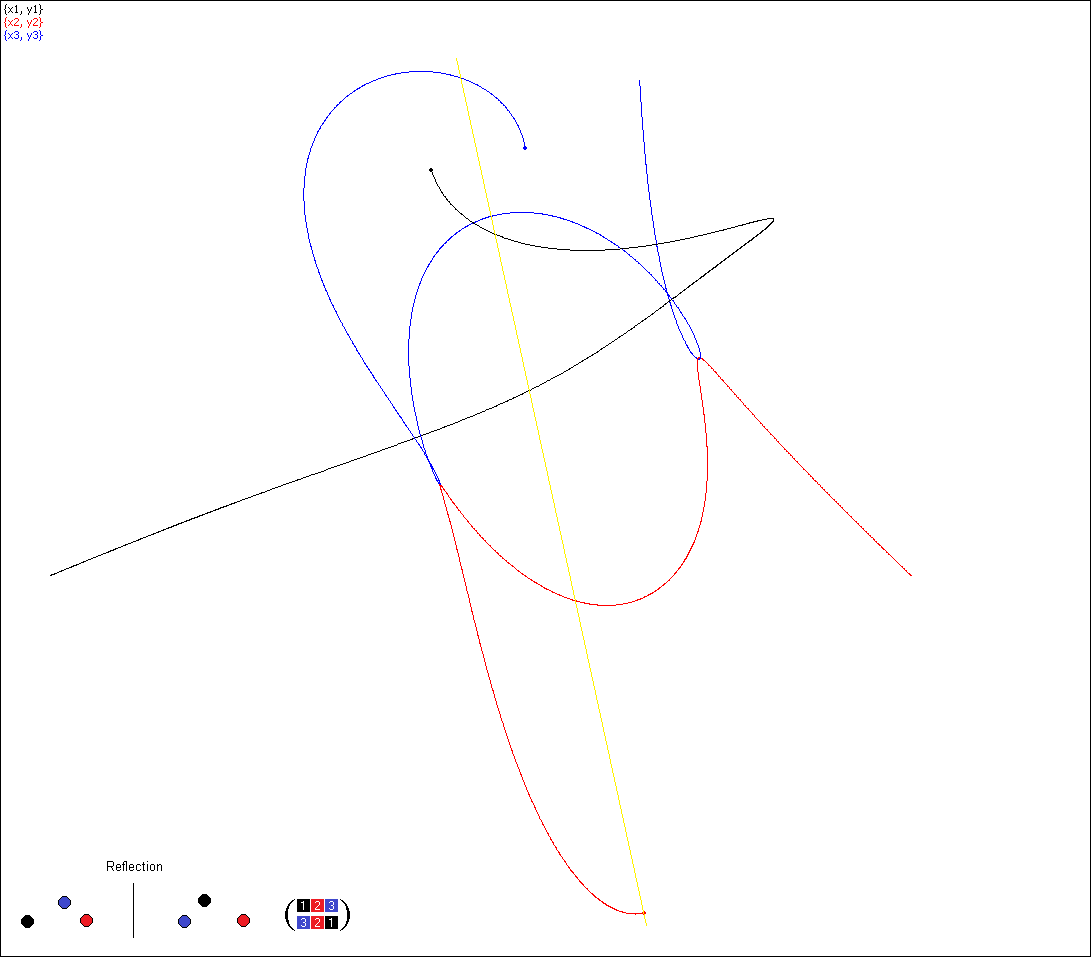Simulation 4. At the moment T/4 the bodies are in an isosceles formation (rather than in syzygy).
The yellow line is the axis of symmetry.Simulation 6, permutation (321)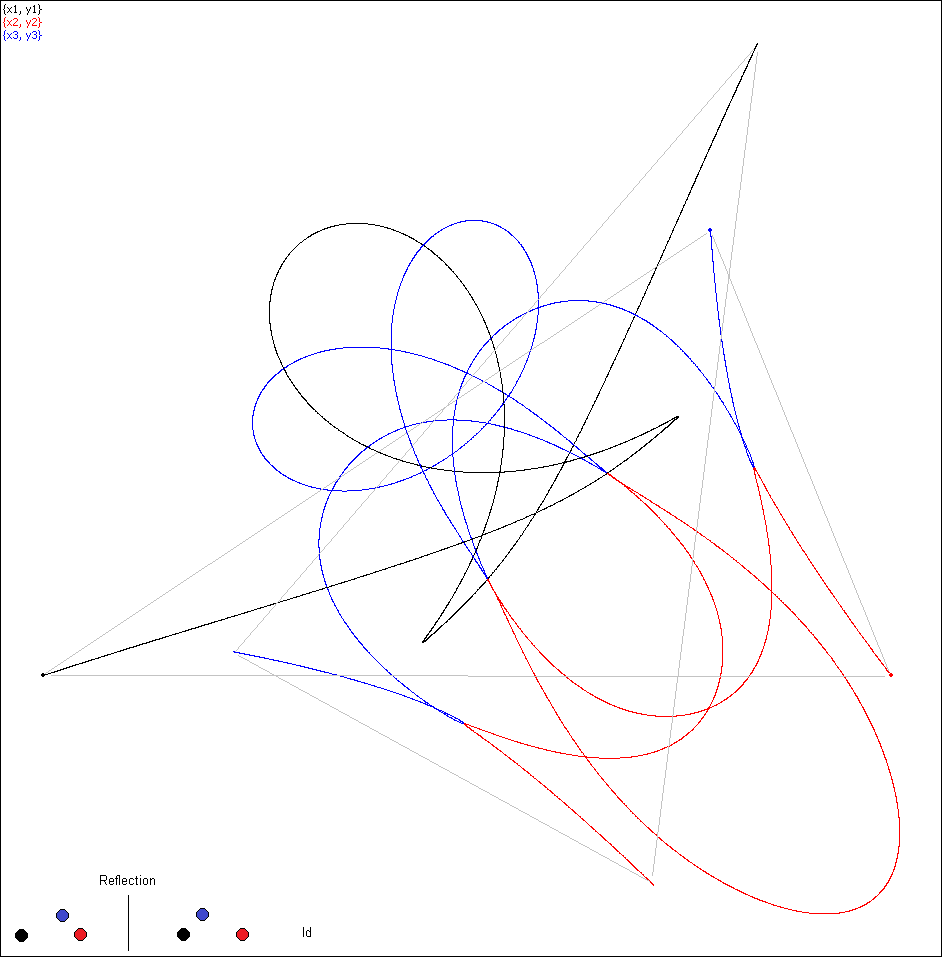Simulation 8, no permutation (Id)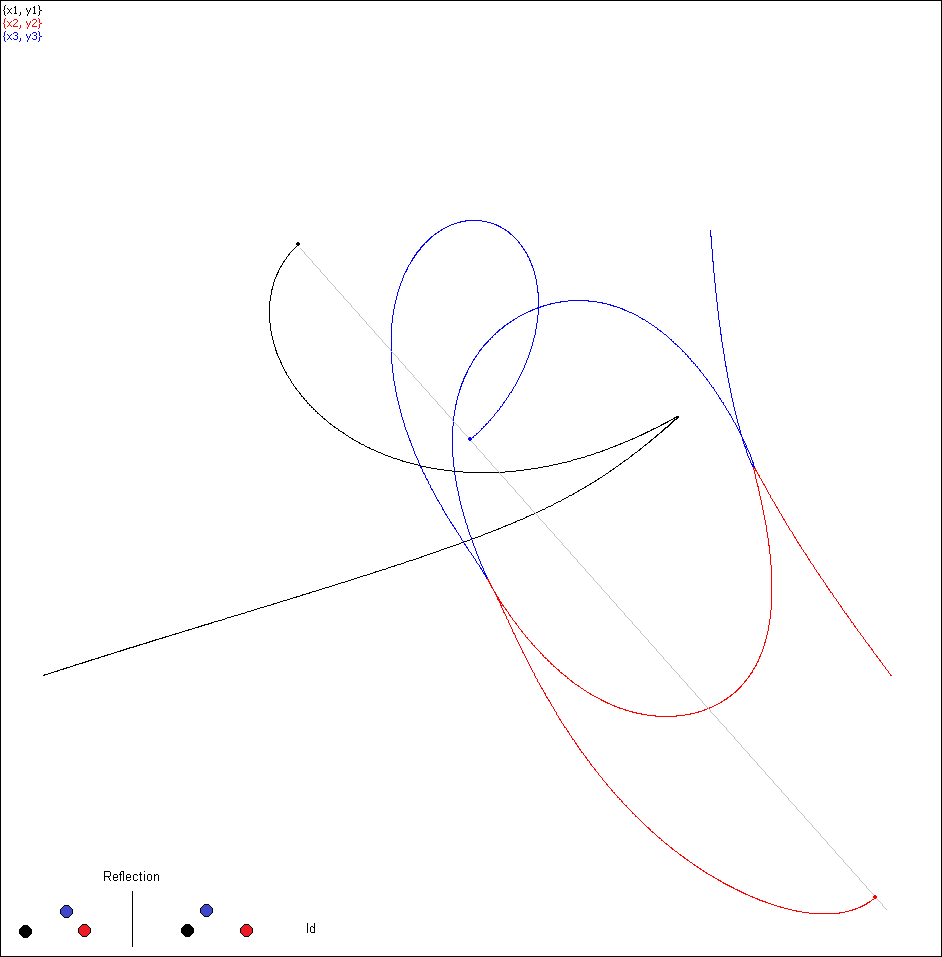Simulation 8. At the moment T/4 the bodies are in syzygy, rather than in an isosceles formation.
The line of syzygy (in gray) is also the axis of symmetry.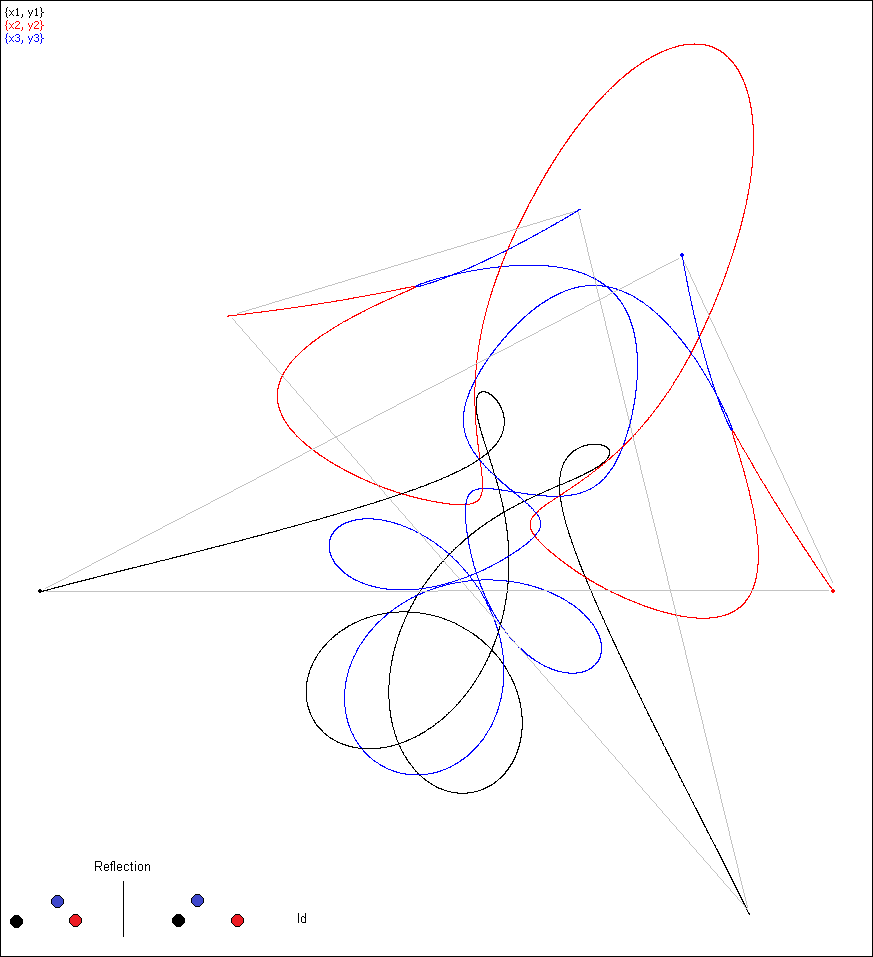Simulation 10, no permutation (Id)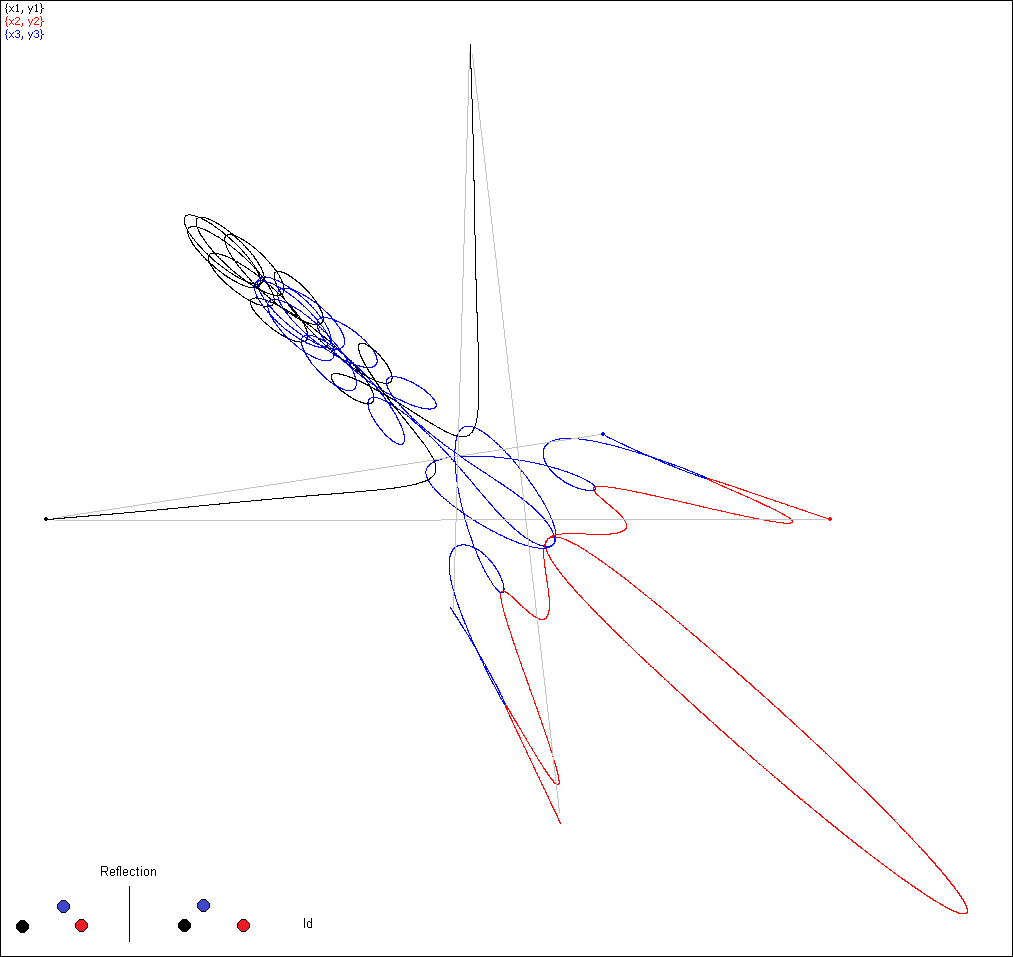Simulation 18, no permutation (Id)Simulation 18: no permutation so that the bodies are in syzygy at T/4 which is also a line of symmetry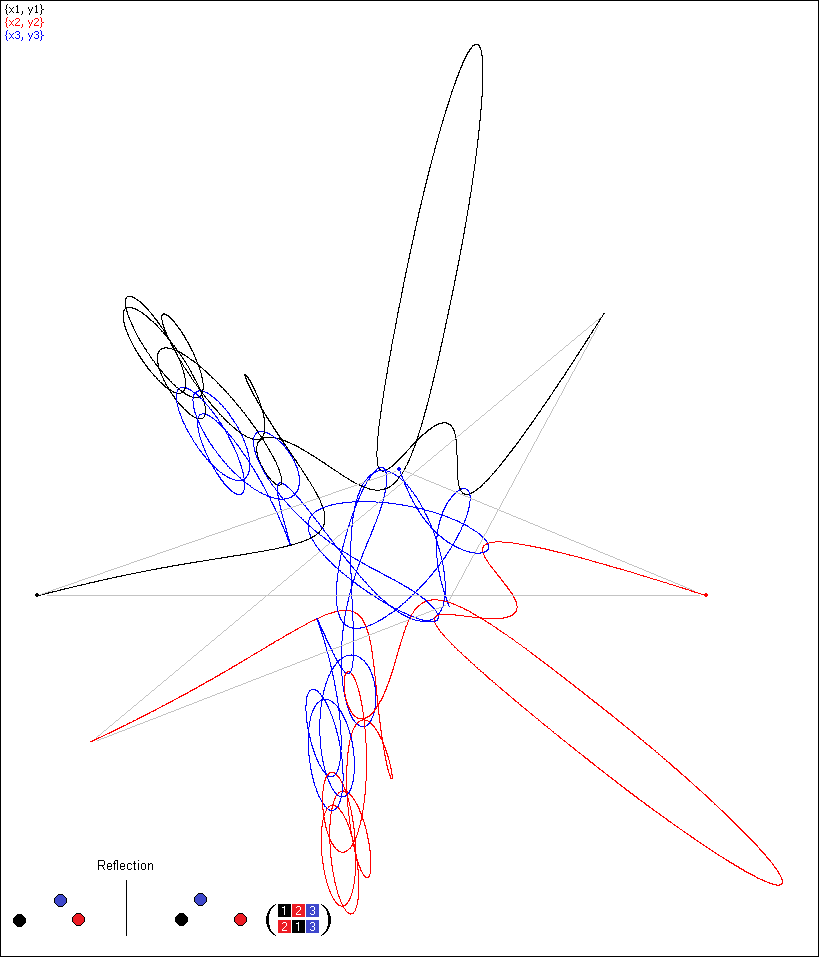Simulation 19, permutation (213)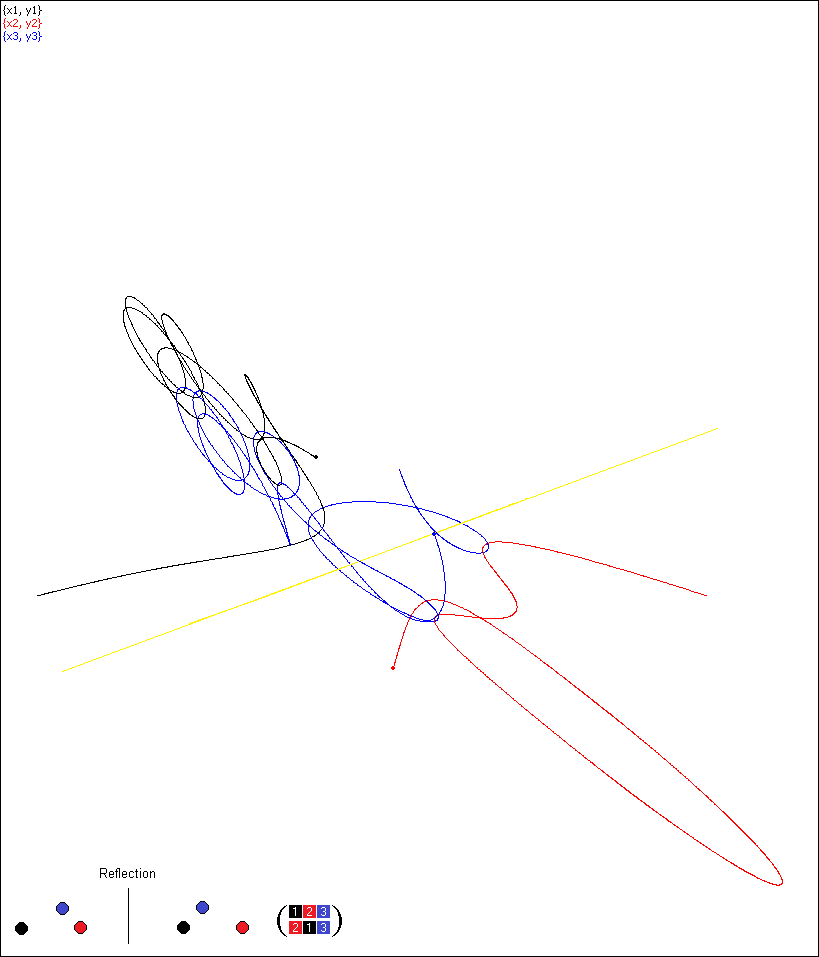Simulation 19, permutation (213)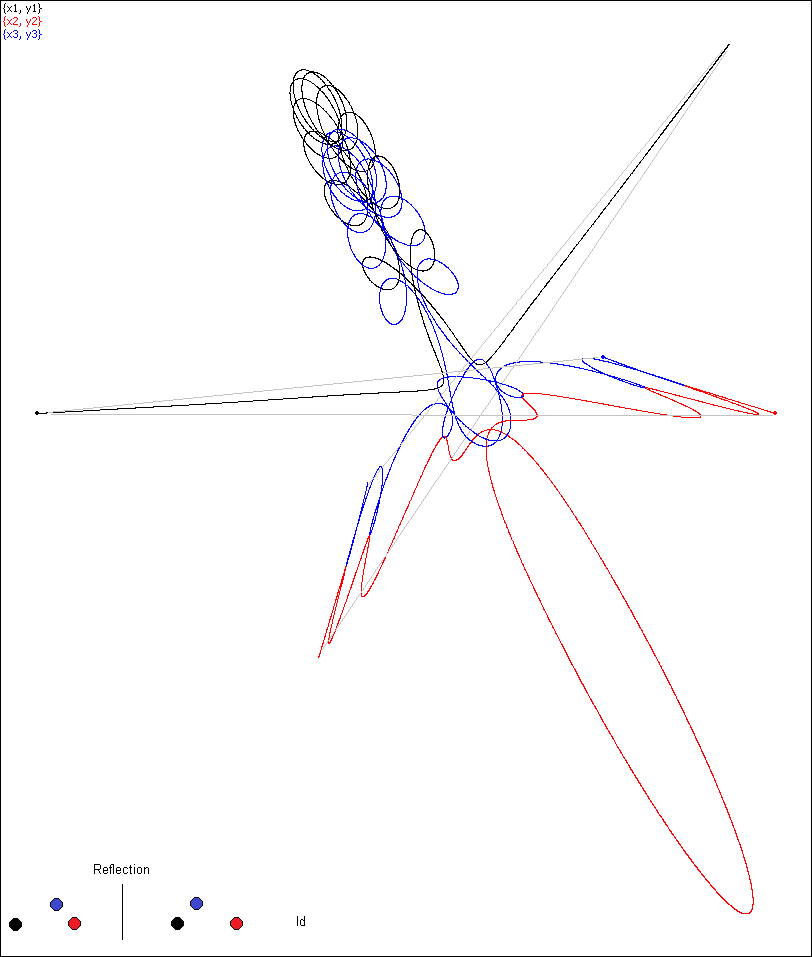Simulation 22, no permutation (Id)Simulation 22,  no permutation (Id). The bodies are in syzygy at T/4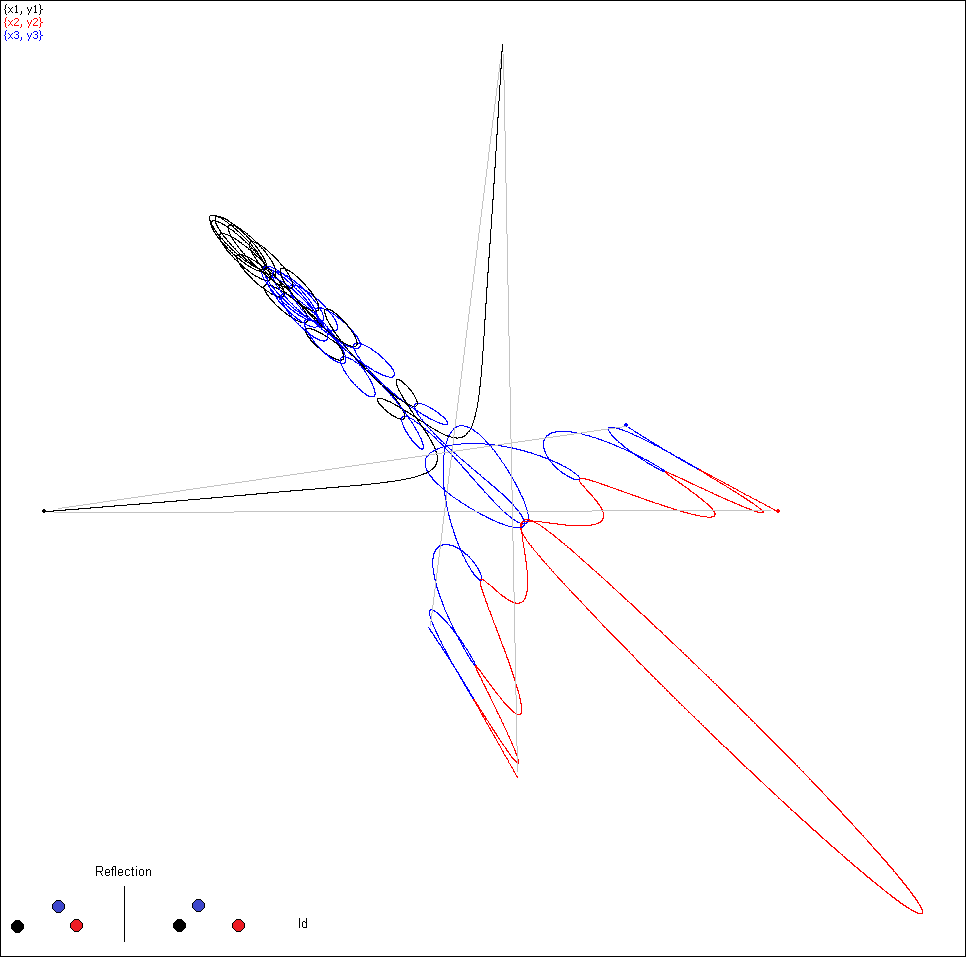Simulation 25, no permutation (Id).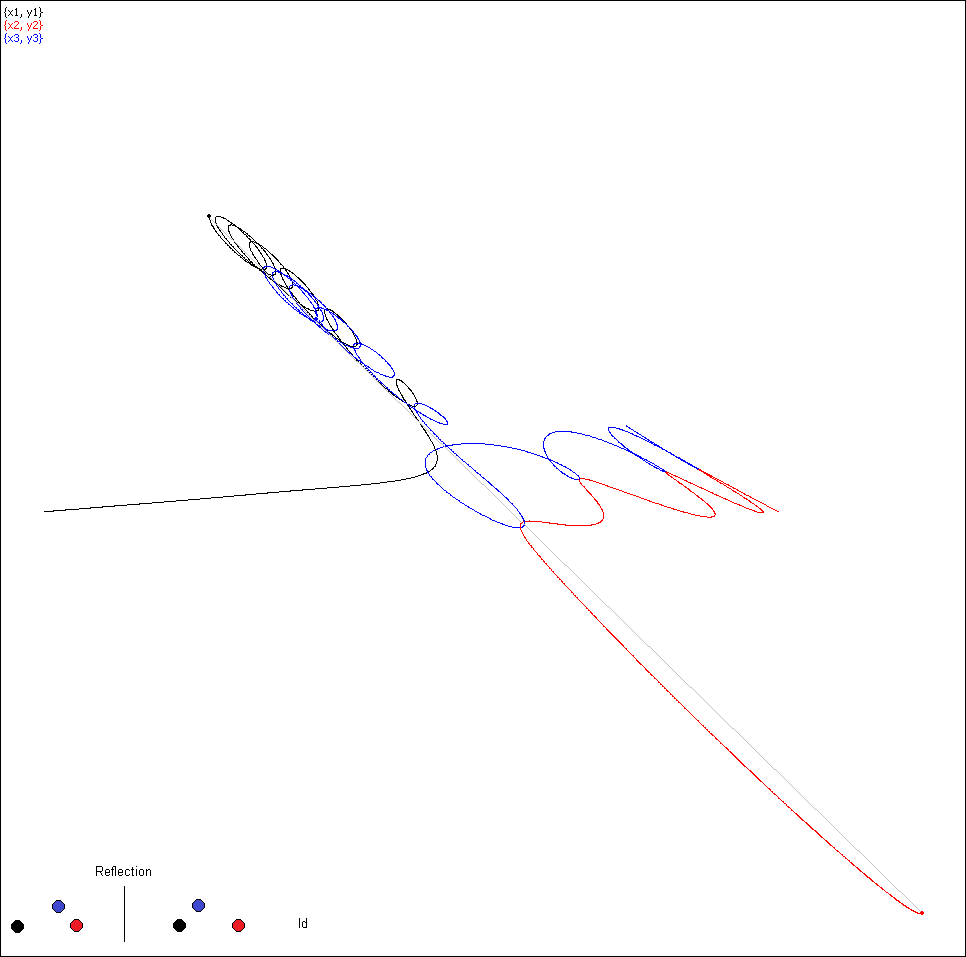Simulation 25, no permutation (Id). The bodies are in syzygy at T/4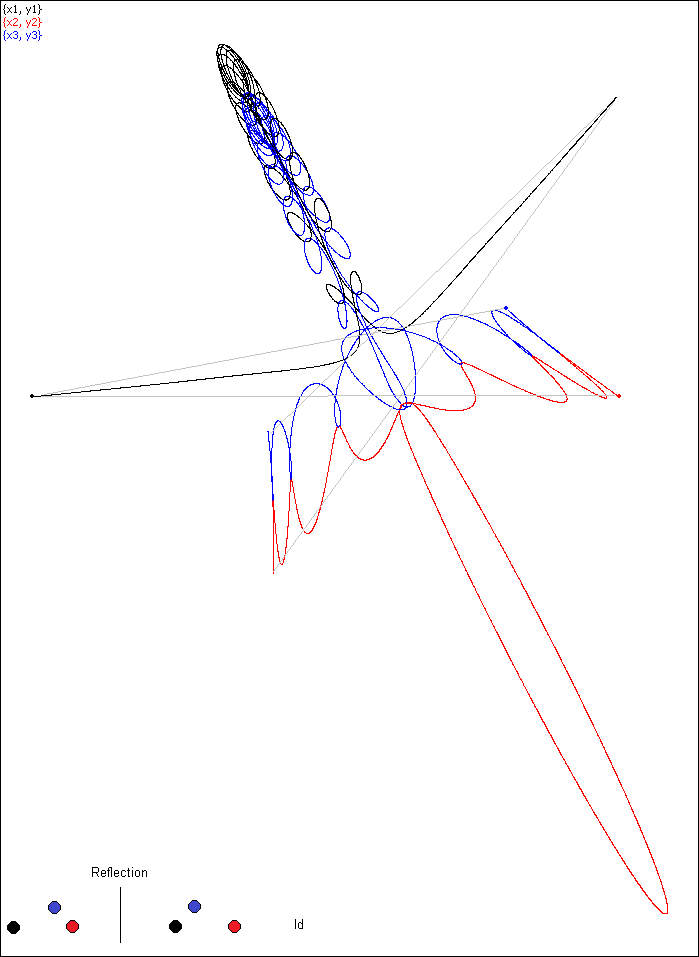Simulation 29, no permutation (Id)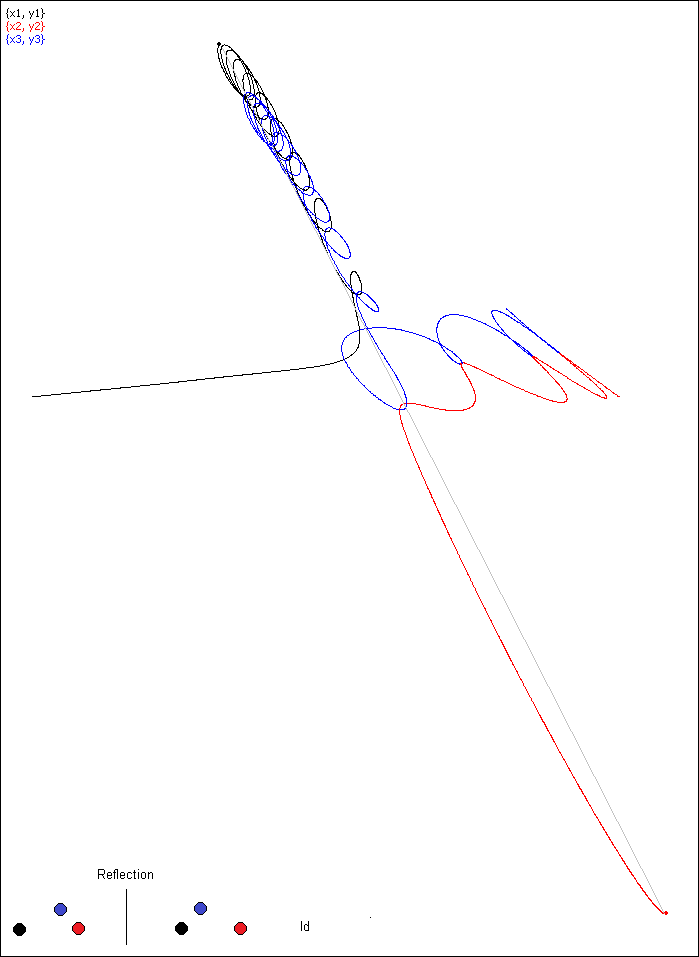Simulation 29, no permutation (Id). The bodies are in syzygy at T/4

3 cases of congruence
with central symmetry, 180˚rotation, and non-Id permutation
so that at t=T/4 a syzygy takes place, one of the bodies being in the middle.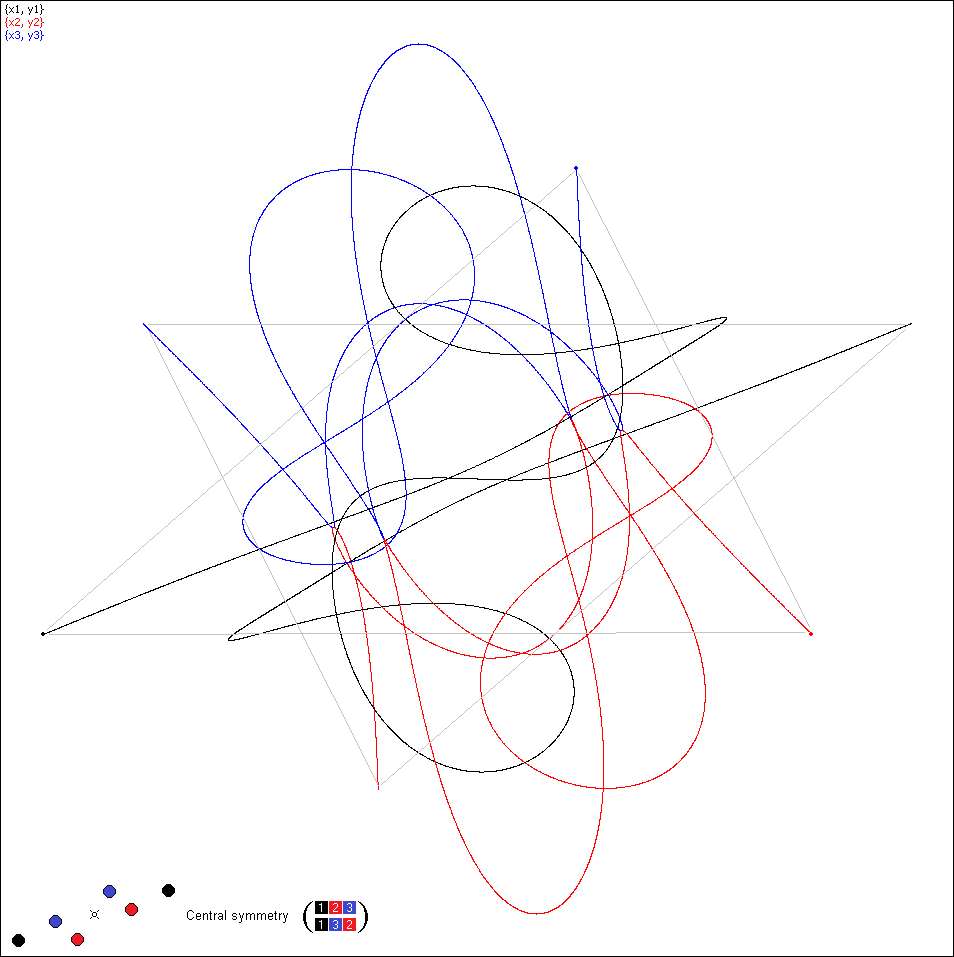Simulation 14, permutation (132)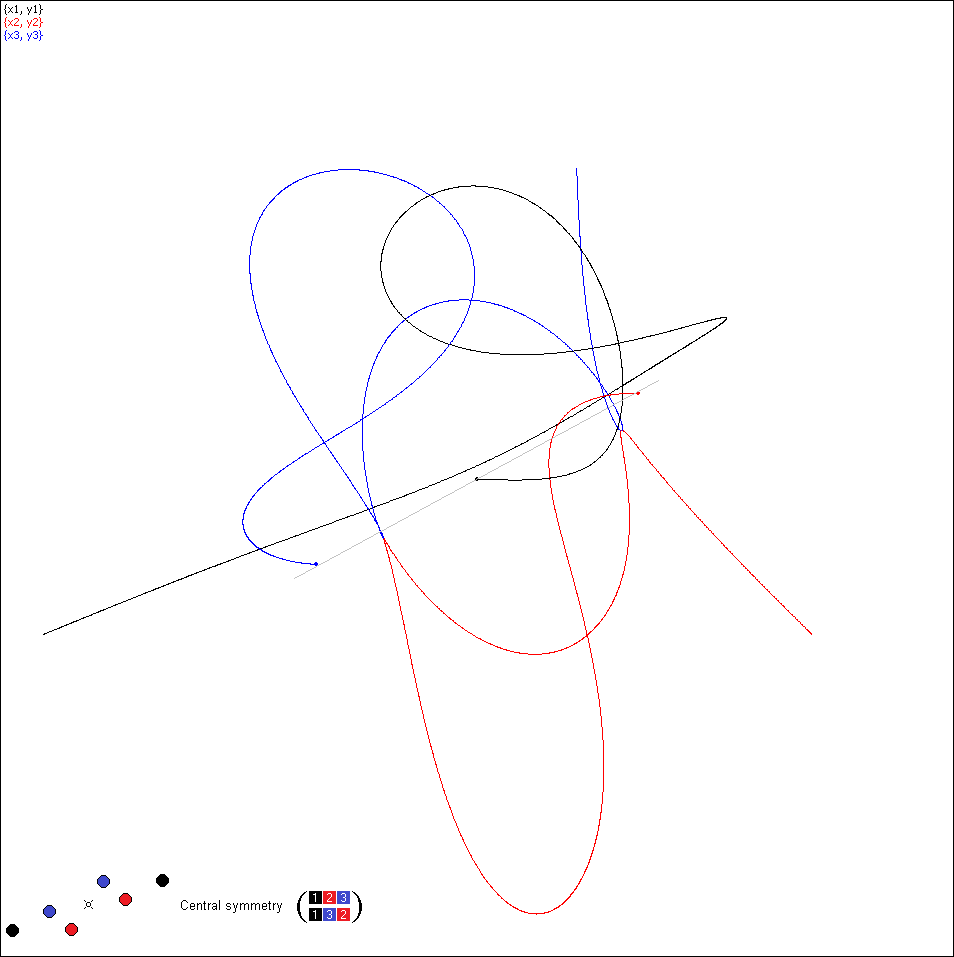Simulation 14 at the moment T/4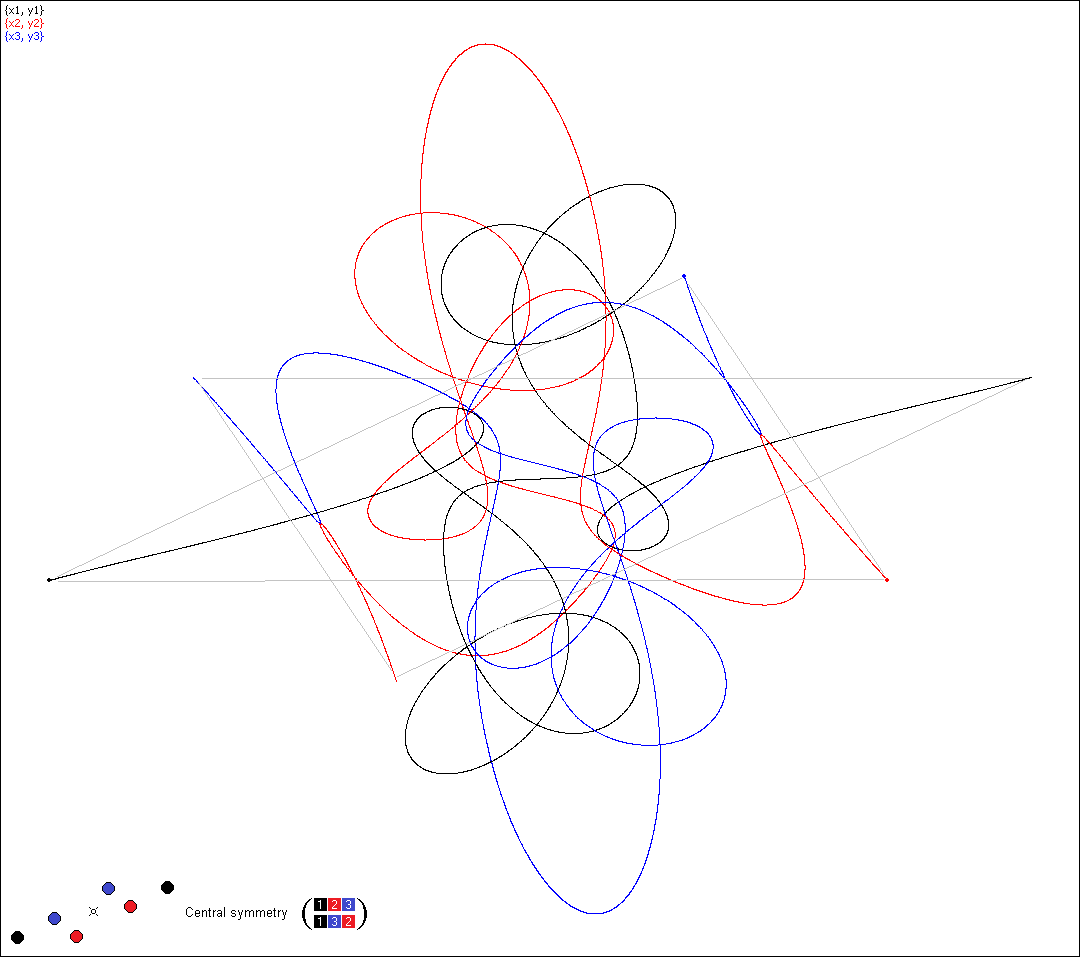Simulation 15, permutation (132)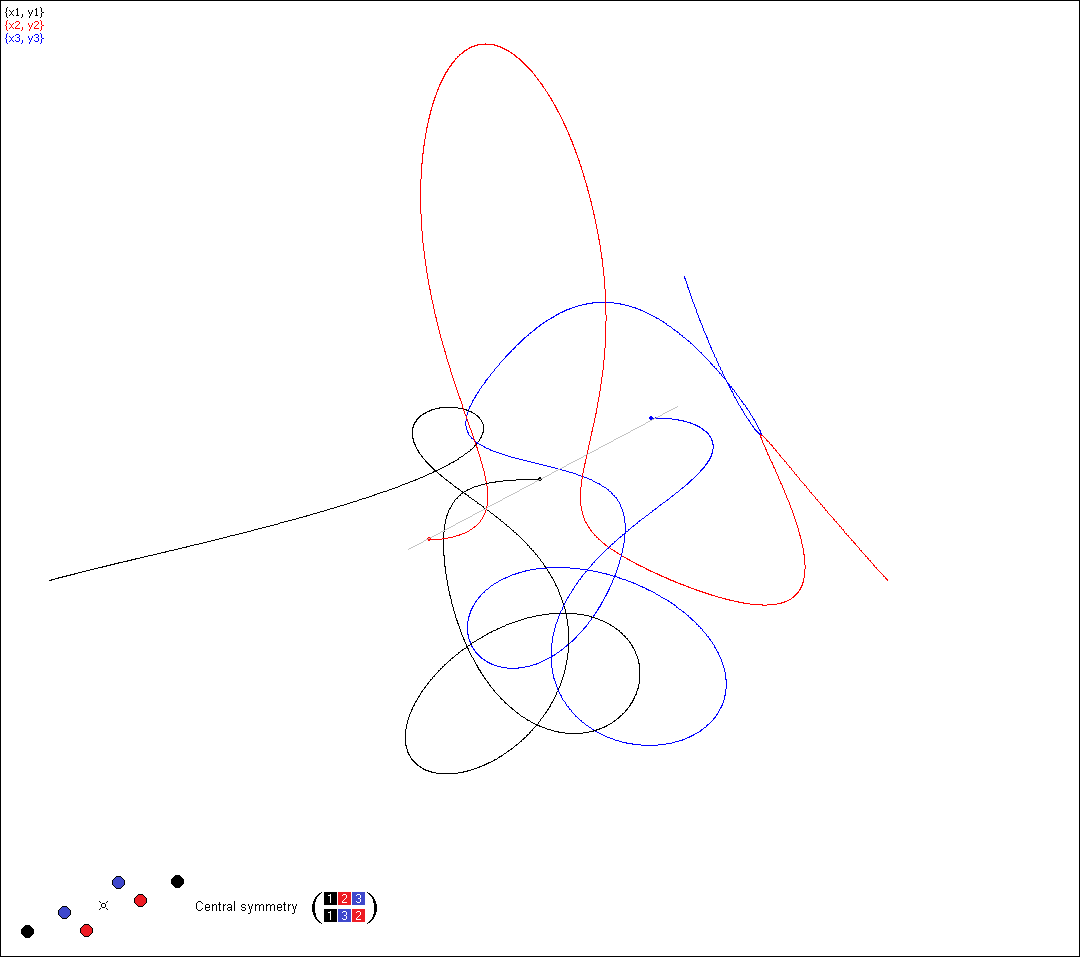Simulation 15 at the moment T/4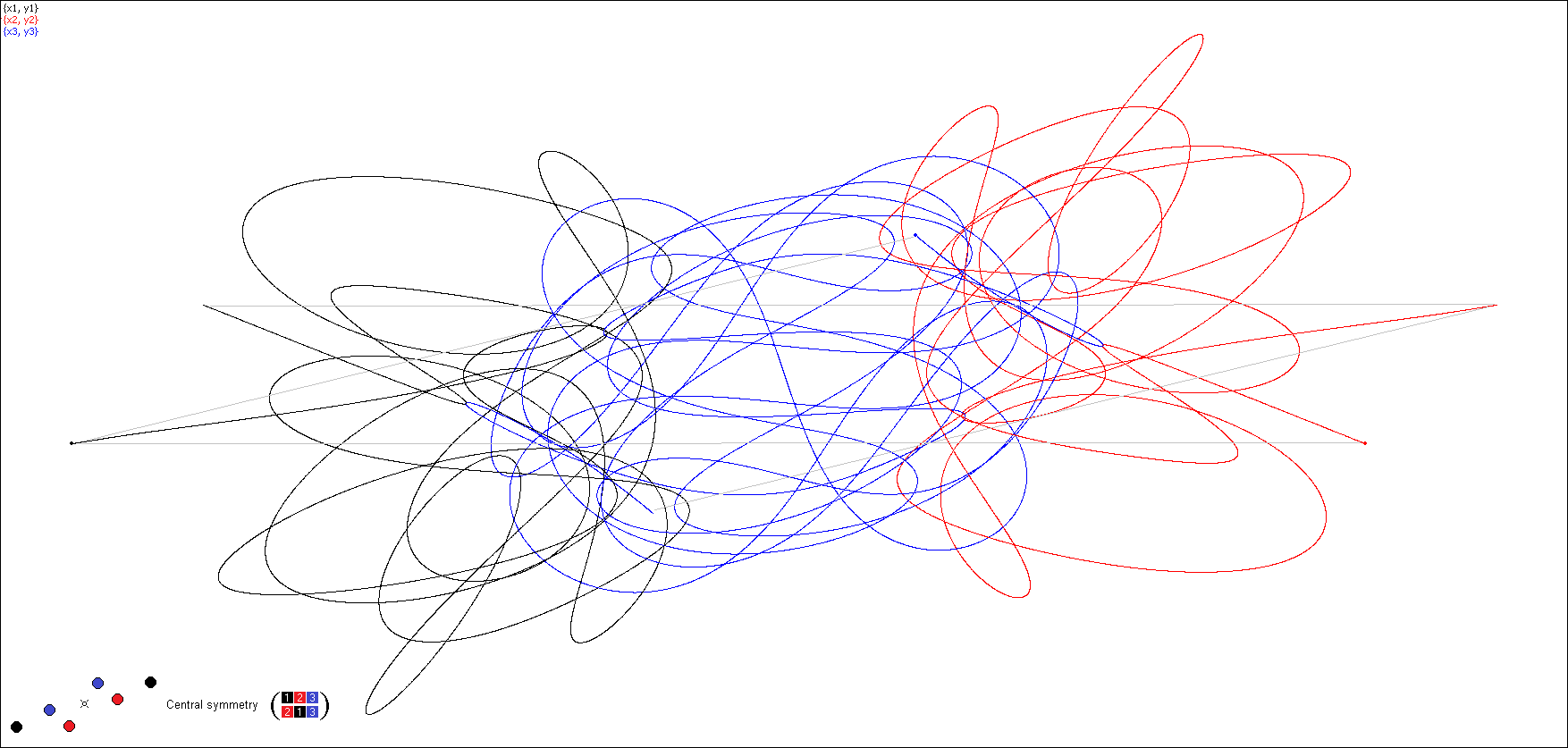Simulation 27, permutation (213)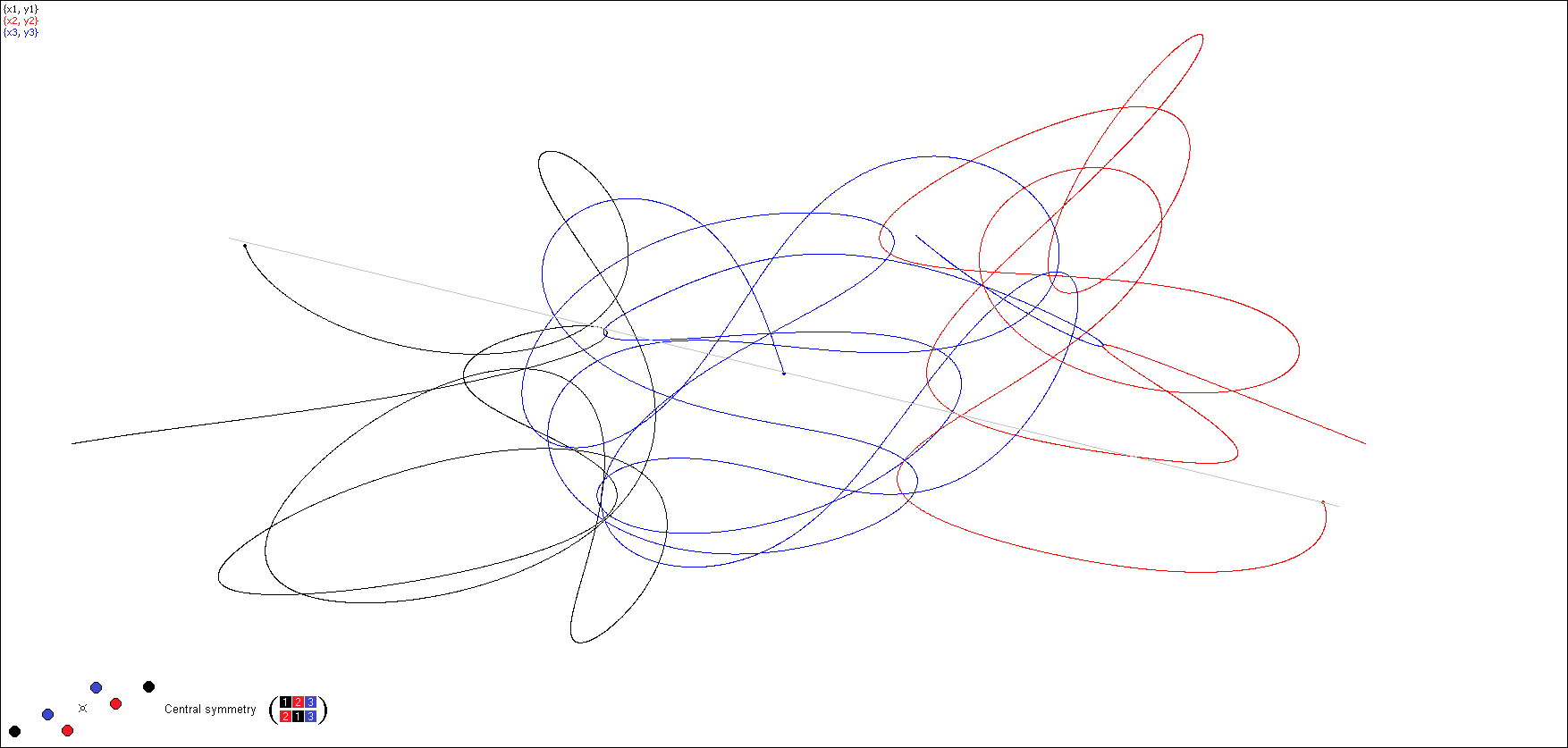Simulation 27 at the moment T/4

4 cases with masses 1, 0.8, 0.8

 At  t = T/2 At  t = T/4 # Congruency Parallel edges Symmetry Permutation Isosceles Syzygy 1 Yes Reflection Id Yes 2 ... 23 24 Reflection Id Yes 25 26 Yes Reflection (132) Yes 27 Yes Reflection Id Yes 28 29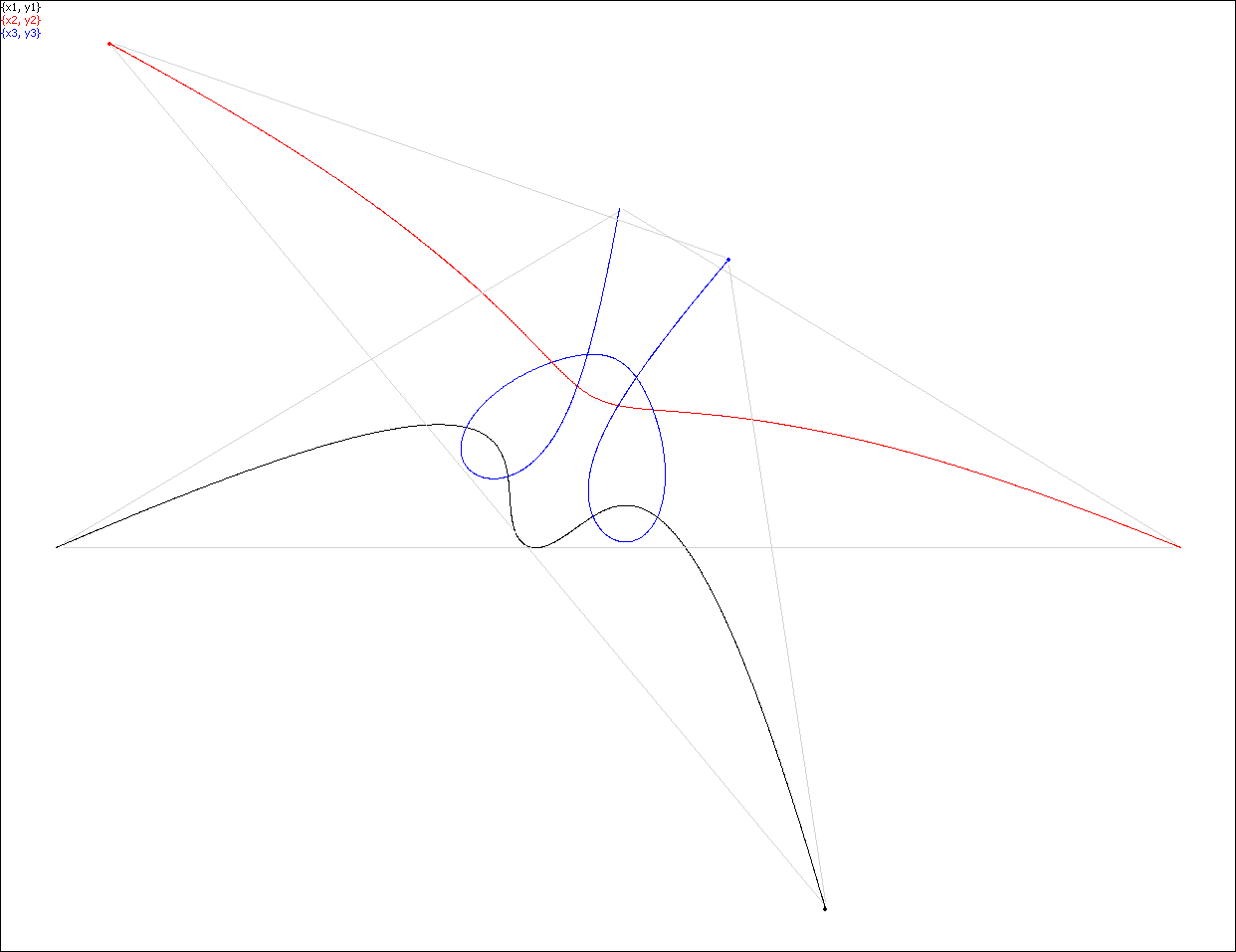Simulation 1. No permutation.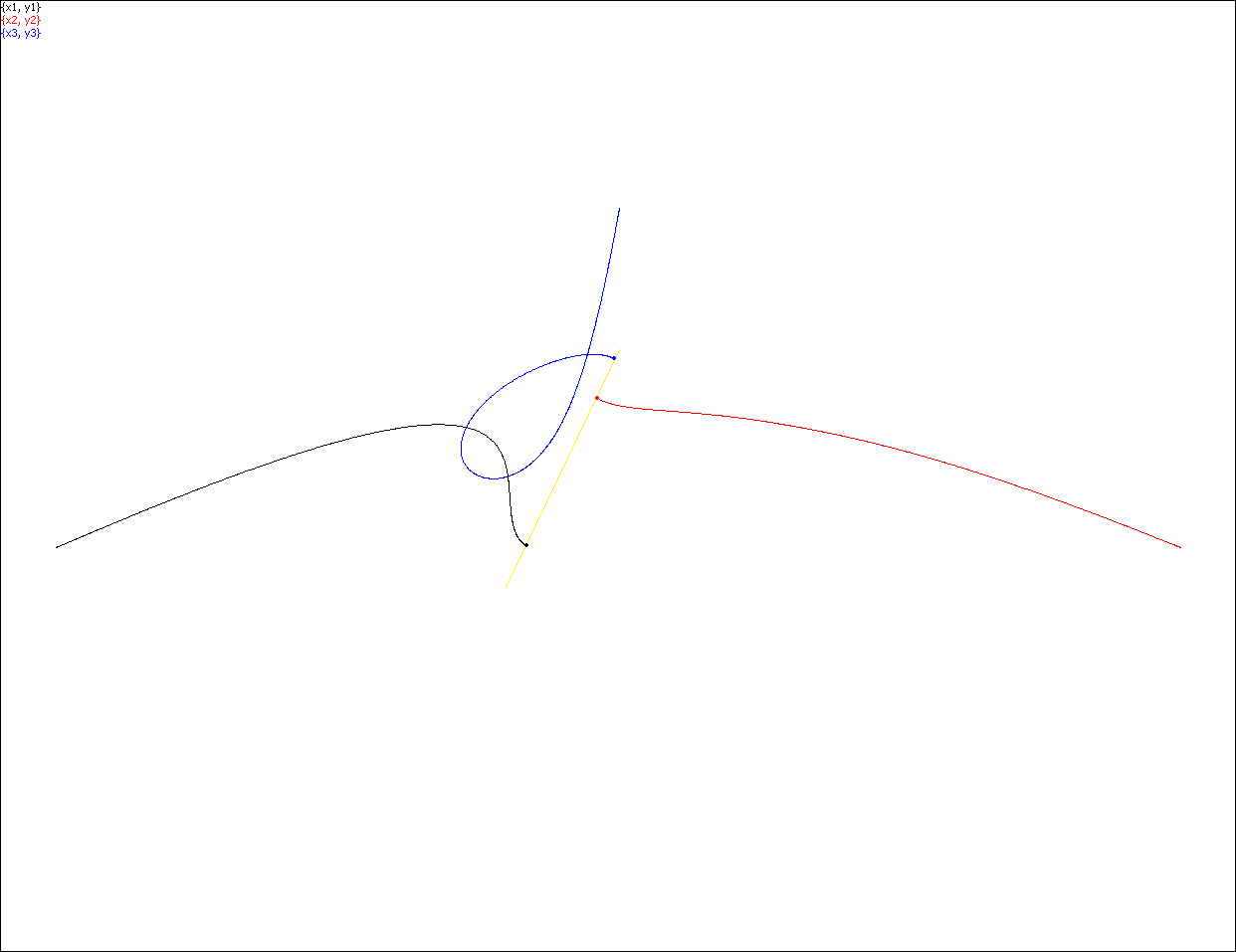Simulation 1. At the moment T/4 the bodies are in syzygy.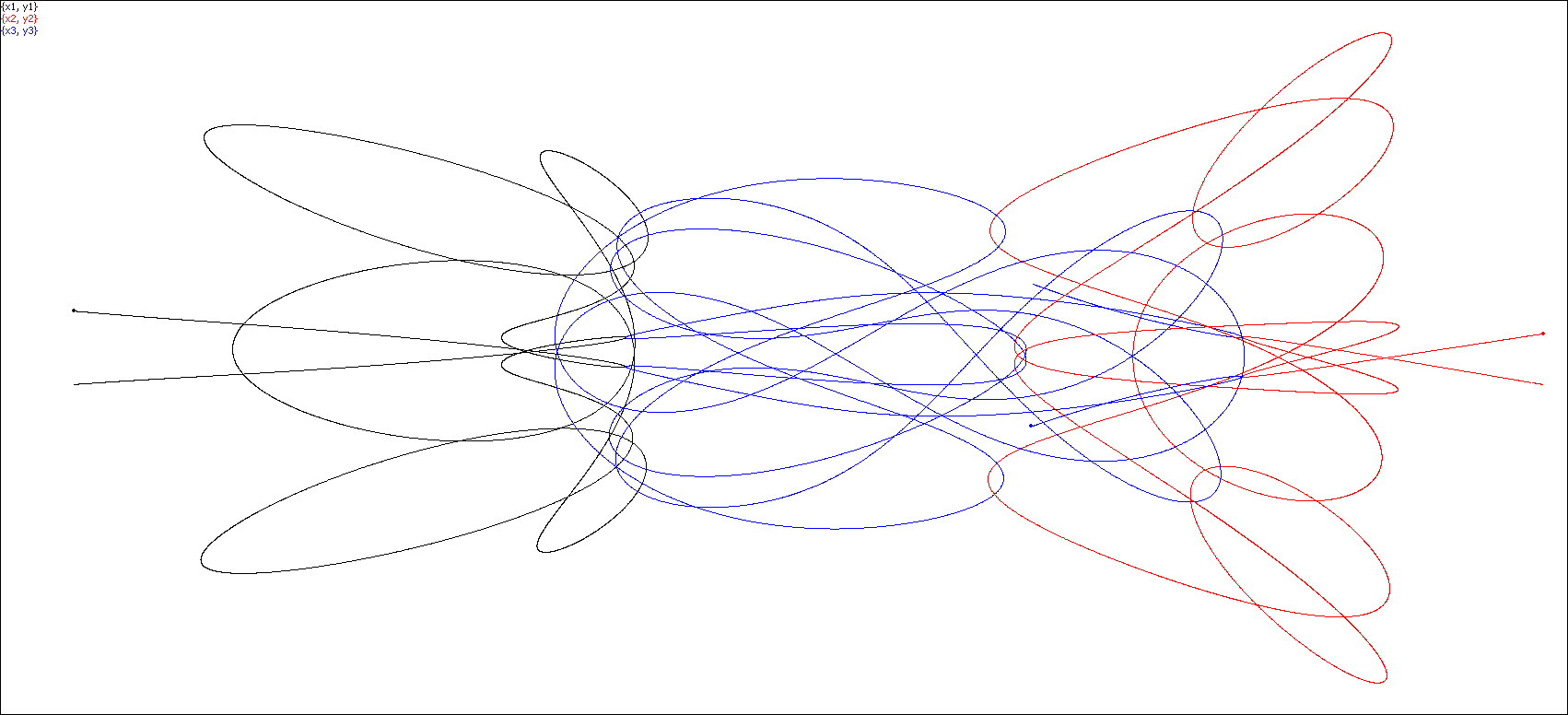Simulation 24. No permutation.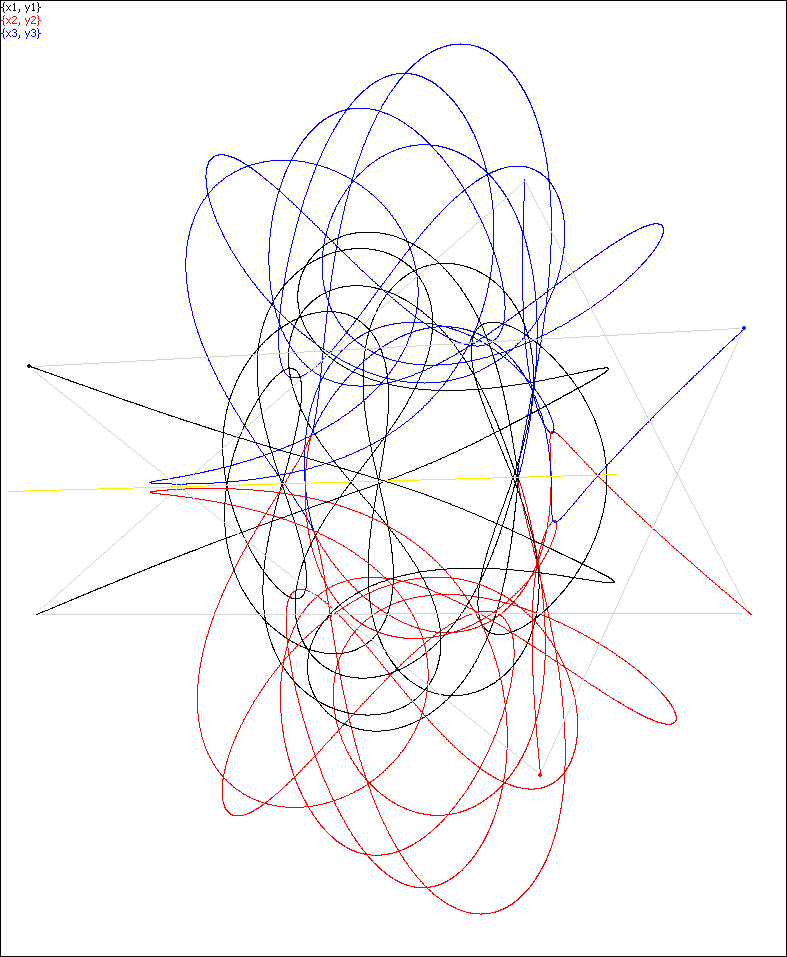Simulation 26. Permutation  (132)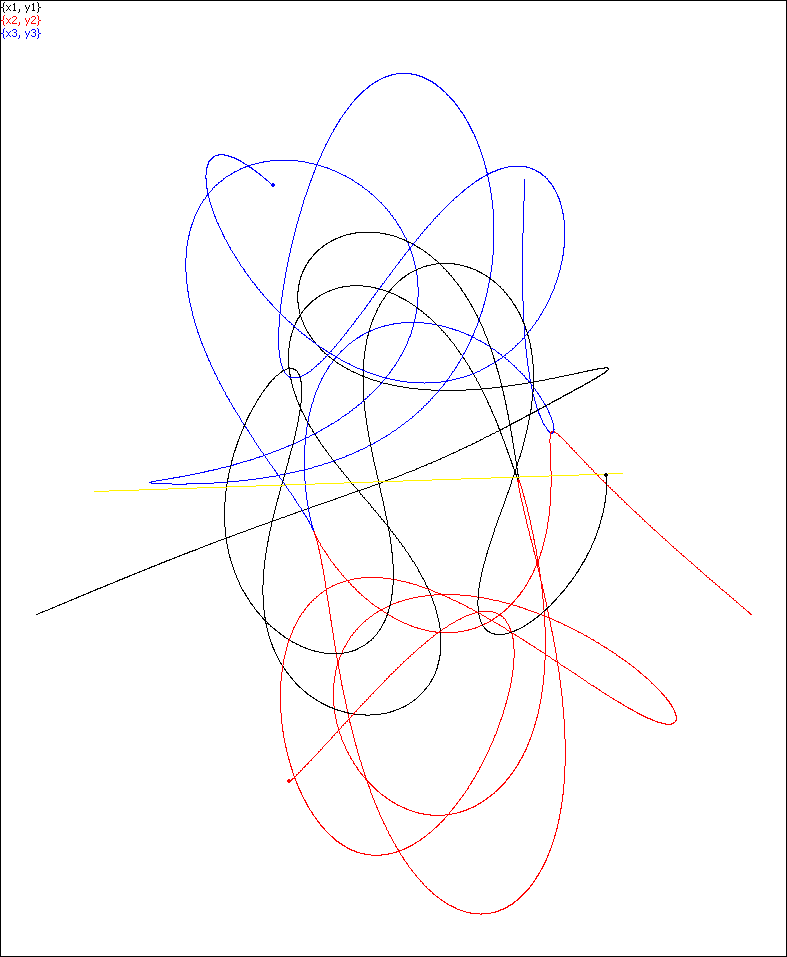Simulation 26. At the moment T/4 the bodies are in an isosceles formation (rather than in syzygy).
The yellow line is the axis of symmetry.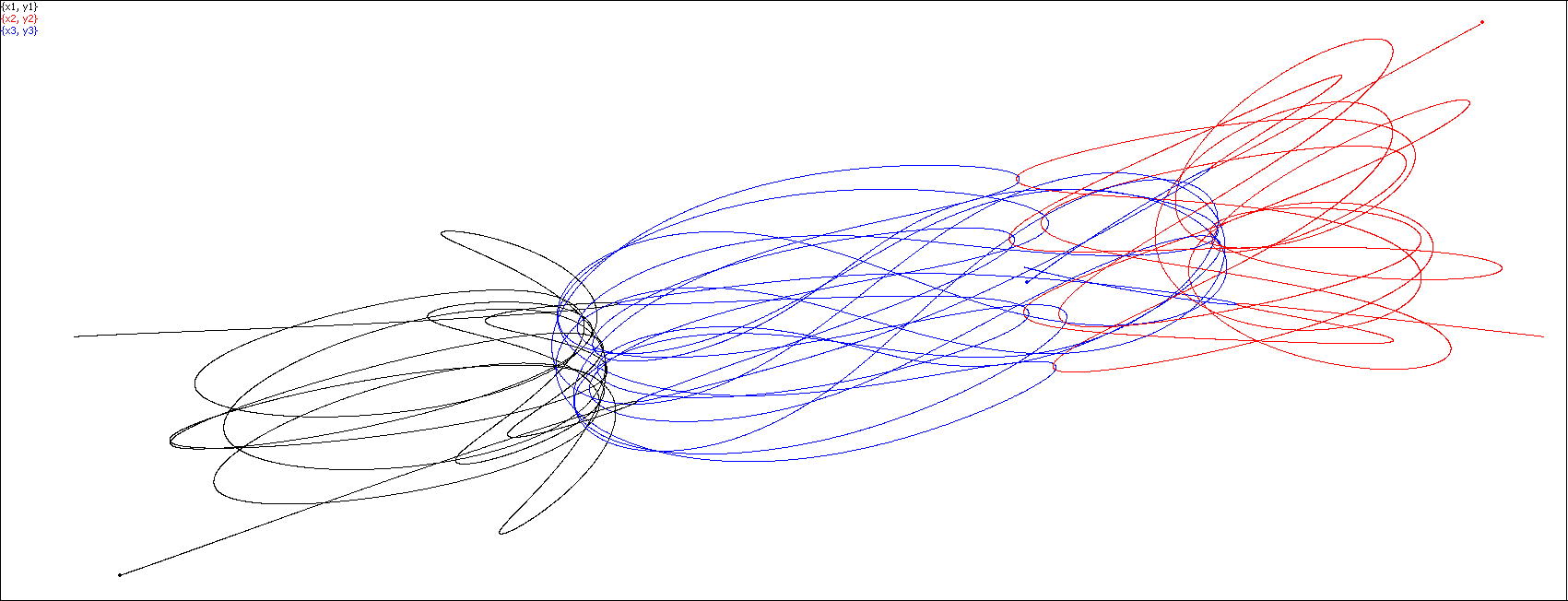Simulation 27. No permutation.